#### Vol. 36, No. 2, 1971

 Download this articleFor screen For printingRecent Issues Vol. 325: 1  2 Vol. 324: 1  2 Vol. 323: 1  2 Vol. 322: 1  2 Vol. 321: 1  2 Vol. 320: 1  2 Vol. 319: 1  2 Vol. 318: 1  2Online Archive Volume: Issue:The Journal Subscriptions Editorial Board Officers Contacts Submission Guidelines Submission Form Policies for Authors ISSN: 1945-5844 (e-only) ISSN: 0030-8730 (print) Special Issues Author Index To Appear Other MSP Journals
Spectral theory of monotone Hammerstein operators

### Charles Vernon Coffman

Vol. 36 (1971), No. 2, 303–322
##### Abstract

Consider the linear integral equation,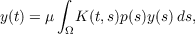(1)

where K(s,t) is a real-valued symmetric positive definite kernel and p(s) is a positive function. Let L denote the inverse of the integral operator u Ω K(,s)u(s)ds, and for a function y in the domain of L,y0, (all functions are assumed to be real valued) define the Rayleigh quotient J(y) for (1) by,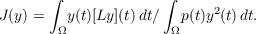If y10 and y1 is in the domain of L and if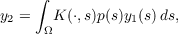Shen several applications of the Schwarz inequality show that,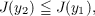with equality only if y1 is an eigenfunction of (1). On the basis of this fact, when the integral operator in (1) is compact, one can develop the complete spectral theory of (1).

In this paper it is shown that the approach indicated above for the study of (1) has a simple and natural extension for the study of the nonlinear integral equation,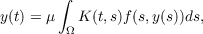(2)

where K(t,s) is as above and f(t,y) is an odd function of y,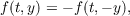and satisfies,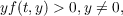and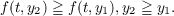Primary: 47.85
Secondary: 45.00
##### Milestones